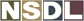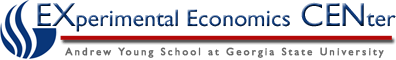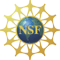Students / Subjects# Network Externalities

(The contents of this page are provided by the Finance and Economics Experimental Laboratory at the University of Exeter.)

Stand-alone demonstration - Try out this multi-player experiment by making decisions against pre-existing results from a real session.

Example subject instructions - View subject instructions.

• Pre-requisite knowledge: ?
• Suitable modules: ?

### Abstract

Students play together in a single large group as consumers who must simultaneously decide whether or not to buy a commodity. The price of the commodity is the same for all and is common knowledge. The value of the commodity to each consumer is equal to that consumer's private value multiplied by the proportion of other consumers who decide to buy. Private values are uniformly distributed across a known interval.

This is an example of a positive network externality, where the entry of additional consumers into a market has a beneficial effect on those who already possess the commodity, e.g. the market for fax machines, which are only useful if there are enough other people you can send a fax to.

### Intended Learning Outcomes

1. Network externalities.

### Discussion of Likely Results

One should only buy the good if the expected value is above the price E[n*V]>=p (where n is the proportion of others buying the good). There are multiple equilibria. One equilibrium has no one buying the good. For instance, if no one buys a fax a machine, then you shouldn't buy a fax machine E[0*V]<p.

In the default setup, the price is £2.40 and the private values are uniformly distributed in the interval [£0.00, £10.00]. Here there is another equilibrium with a positive n. Those deciding to buy the good have should have V such that E[n]V>p. In words, they each decide to buy when for private values above a common threshold value V=p/E[n]. What should that value be? The proportion of others who buy is approximately (10-V)/10, so each consumer is indifferent between buying and not buying when V(10-V)/10=2.4, which has solutions for V=4 and V=6. When V=4, this is the success equilibrium where 60% of the consumers buy the good. When V=6, it is in an unstable equilibria, which one can call the "tipping point". If people with values of 5.9 start buying it then, the object would be a hit, that is, it will go to the equilibrium with V=4. This is because the proportion of those buying it is now (10-5.9)/10=0.41 and p/0.41=2.4/0.41=5.85. So those with values above 5.85 will start buying the good. Repeating this calculation (an infinite number of times) one will arrive at V=4.